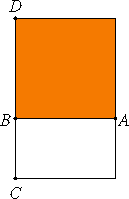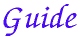# Proposition 20

If a rational area is applied to a rational straight line, then it produces as breadth a straight line rational and commensurable in length with the straight line to which it is applied.

Let the rational area AC be applied to AB, a straight line once more rational in any of the aforesaid ways, producing BC as breadth.I say that BC is rational and commensurable in length with BA.

Describe the square AD on AB. Then AD is rational.

But AC is also rational, therefore DA is commensurable with AC. And DA is to AC as DB is to BC. Therefore DB is also commensurable with BC, and DB equals BA. Therefore AB is also commensurable with BC.

But AB is rational, C therefore BC is also rational and commensurable in length with AB.

Therefore, if a rational area is applied to a rational straight line, then it produces as breadth a straight line rational and commensurable in length with the straight line to which it is applied.

Q.E.D.

###This proposition is a converse of the last, except that it’s preceded by applying an area to a straight line to get the rectangle. That would be more evident if it read “If one side of a rational rectangle is rational, then the other side is rational and commensurable with the first.”

It’s often easier to understand the statement and proof they’re translated into modern language. Let a be the length of AB, and b be the length of BC. Since the rectangle AC is rational, that means its area ab is a rational number. Since AB is a rational line, that means a2 is a rational number. Therefore, a/b is a rational number, that is, lines AB and BC are commensurable. Also, b2 is a rational number, that is, line BC is a rational line.

#### Use of this proposition

This proposition is used frequently in Book X starting with X.26.